Let’s get started.

## Given problem

Given a rotated array, find the index of specific element.

Assuming that, we have a rotated array like the below image: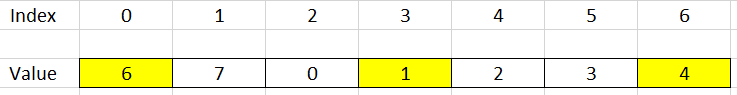How can we find the index of specific element in optimal time?

## Using Linear Search to find element

Normally, the first thing we do is to use brute-force to deal with problem. In this case, we will use linear search to do it.

``````public static int search(int[] nums, int target) {
int pos = 0;
for (int i = 0; i < nums.length - 1; ++i) {
if (nums[i] == target) {
pos = i;
break;
}
}

return pos;
}
``````

The complexity of using Linear Search is:

• Time complexity: O(n)
• Space complexity: O(1)

The drawback of using Linear Search is about the time complexity - O(n). But we can improve it in O(logn), because our rotated array has sub-arrays that are increased array.

So we can use Binary Search. Next, we will analyze our problem when using Binary Search.

1. Case 1 - When we jump into the right sub-arrayBut in this case, we can have two issues:

• our target will under this range.

For example: target = 2, then we have: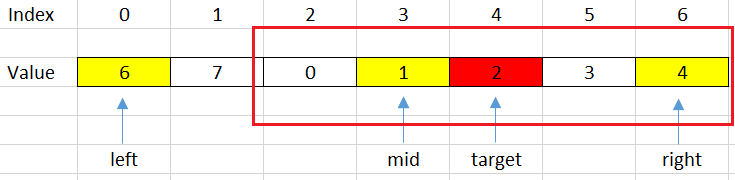Then, we will shift left with steps: `left = mid + 1;`

• our target will not be contain in this range.

For example: target = 7, then we have: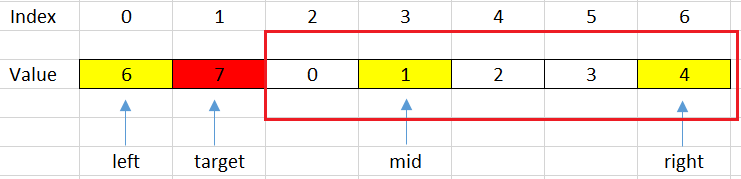Then, we will shift right with steps: `right = mid - 1;`

Based on the above conditions, we can have the segment code:

`````` if (nums[mid] <= nums[right]) {
if (nums[mid] < target && target <= nums[right]) {
left = mid + 1;
} else {
right = mid - 1;
}
}
``````
2. Case 2 - When we jump into the left sub-array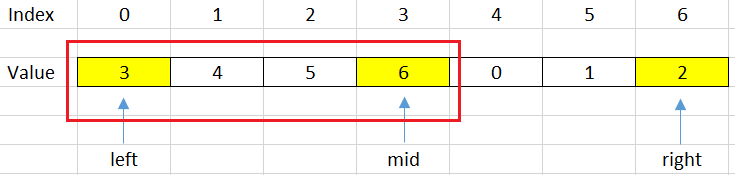But in this case, we also have two issues:

• our target will under this range.

For example: target = 4, it can be described in a below image.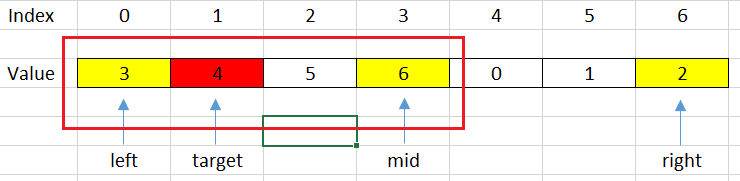Then, we will shift left with some steps: `right = mid - 1;`

• our target will be not contain in this range.

For example, target = 1, then we have: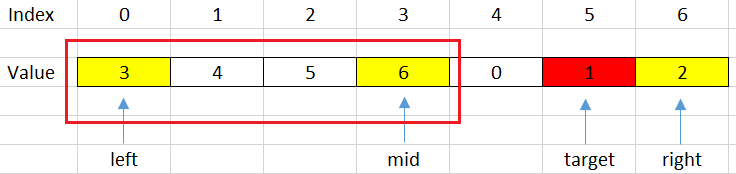Then, we will shift right with some steps: `left = mid + 1;`

Based on above conditions, we can some code to describe it.

`````` if (nums[mid] >= nums[left]) {
if (nums[left] < target && target < nums[mid]) {
right = mid - 1;
} else {
left = mid + 1;
}
}
``````

Below is our source code of this way:

``````public static int search(int[] nums, int target) {
int left = 0;
int right = nums.length - 1;

while (left <= right) {
int mid = left + (right - left) / 2;
if (nums[mid] == target) {
return mid;
}

if (nums[mid] <= nums[right]) { // right half is sorted
if (target > nums[mid] && target <= nums[right]) {
left = mid + 1;
} else {
right = mid - 1;
}
} else {        // left half is sorted
if (nums[left] <= target && target < nums[mid]) {
right = mid - 1;
} else {
left = mid + 1;
}
}
}

return -1;
}
``````

The complexity of Binary Search is:

• Time complexity: O(log(n)).
• Space complexity: O(1).

## Wrapping up

• Understanding why we can apply Binary Search in this problem.

• Go from the brute-force solution, then optimize it.